Search test library by skills or roles
⌘ K

Here are some sample NumPy questions from our premium questions library (10273 non-googleable questions).

### Skills

#### Accounting

🧐 Question

Medium

Array Manipulation and Summation
Array Manipulation
Mathematical Operations
Solve
`Consider the following code snippet:`
`What will be the value of G after executing the code?`

Medium

Matrix Eigenvalues and Diagonalization
Linear Algebra
Matrix Operations
Solve
`Consider the following code snippet:`
`After running this code, which of the following statements is true regarding the B matrix?`
🧐 Question🔧 Skill

Medium

Array Manipulation and Summation
Array Manipulation
Mathematical Operations
2 mins
NumPy
Solve

Medium

Matrix Eigenvalues and Diagonalization
Linear Algebra
Matrix Operations
3 mins
NumPy
Solve
🧐 Question🔧 Skill💪 Difficulty⌛ Time
Array Manipulation and Summation
Array Manipulation
Mathematical Operations
NumPy
Medium2 mins
Solve
Matrix Eigenvalues and Diagonalization
Linear Algebra
Matrix Operations
NumPy
Medium3 mins
Solve

## Adaface questions are trusted by enterprises globally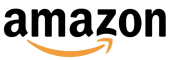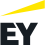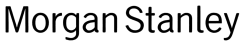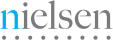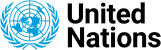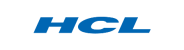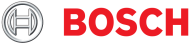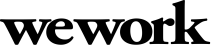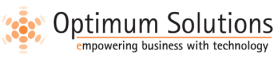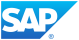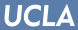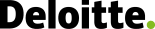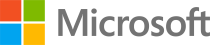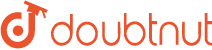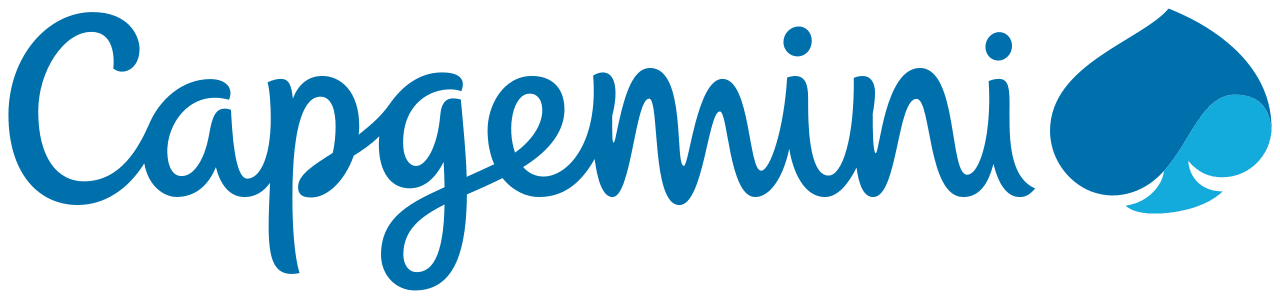We evaluated several of their competitors and found Adaface to be the most compelling. Great default library of questions that are designed to test for fit rather than memorization of algorithms.

Swayam Narain, CTO, AffableJoin 1200+ companies in 75+ countries.
Try the most candidate friendly skills assessment tool today.